Portfolio Boss Documentation

# Moving Average Persistence Rule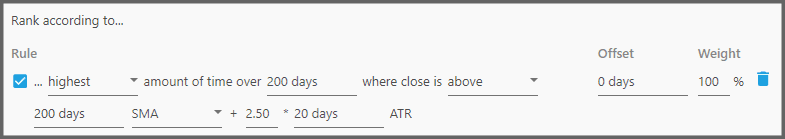This rule ranks the instruments based on their persistence in staying inside the specified zone (habitat), as explained in the Moving Average Persistence Filter page.

1.  The first parameter defines how the sorting happens.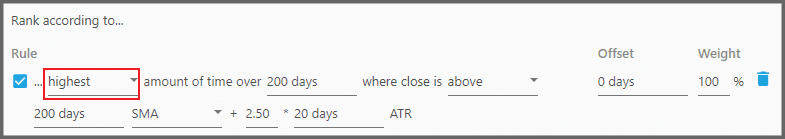“Highest” means the instruments with greater persistence (percentage) are ranked higher. While “Lowest” means you're looking for the least persistent ones.

2.  The second parameter defines the period of days where persistence is calculated.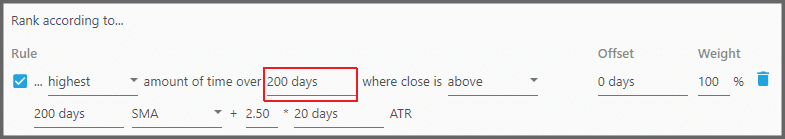For example as shown in the screenshot above, if the instrument stayed 200 days in the specified habitat, out of the 200 days specified here, its persistence would be 100%.

3.  The third parameter defines the habitat where the instrument must stay.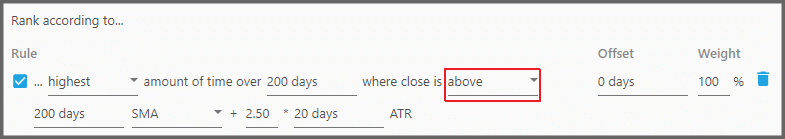“Above” means the area above the separator line (i.e. above the Moving Average plus the Multiplied ATR). “Below” means the area below the separator line. And “Between” means the area between the Moving Average and the separator line.

4.  The fourth parameter defines the Moving Average period.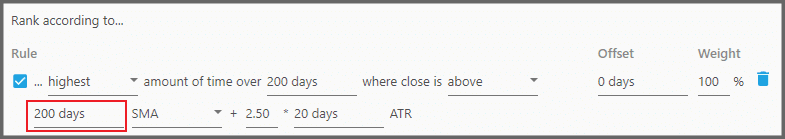The usual values are 26, 50, or 200 days.

5.  The fifth parameter defines the type of Moving Average used.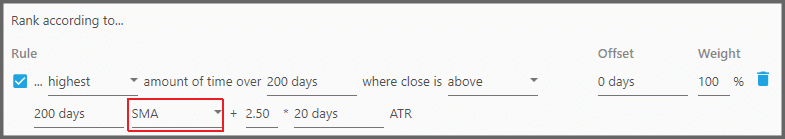You may choose either SMA (Simple Moving Average) or EMA (Exponential Moving Average).

6.  The sixth parameter defines the ATR multiplier.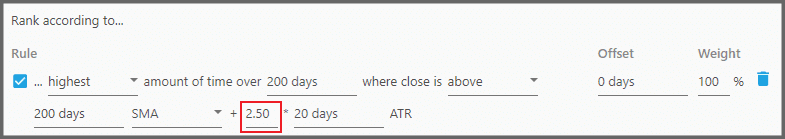It lets you adjust how far the habitat separator is located from the Moving Average line.

7.  The seventh parameter defines the ATR period.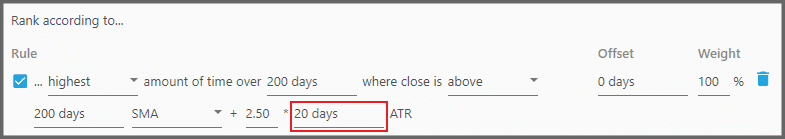The usual values are 9, 10, 14, or 20 days.